(User Defined Functions (UDFs## (User Defined Functions (UDFs

بازدید : 508 پنجشنبه 11 دي 1393

## (User Defined Functions (UDFs

Overview of User Defined Functions
The user defined function table
Format of the function data transfer file
Format of the function result file
Example: Linear Regression Function

Users may write their own grads functions in the computer language of their choice, and have them available from the grads expression facility (via the `display` command). Some possible user defined functions might be:
• filtering functions
• grid interpolation functions
• thermodynamic functions

You may write a function that can be invoked via the grads expression facility. This function may be written in any computer language, and may perform any desired I/O, calculations, etc. Please read the following documentation carefully to understand the restrictions to this capability.

### Overview of User Defined Functions

The steps that grads uses to invoke a user defined function are:

1. When grads is first started, it reads a file that describes the user defined functions. This file is called the 'user defined function table'.

2. When a user function is invoked via the display command expression, GrADS parses the arguments to the functions, obtains the results of any expressions, and writes the resultant data to a 'function data transfer file'.

Please note that in a user-defined function adding the double quote ("") around a `char` argument passes the string directly without the usual conversion to lower case and removal of blanks, e.g.,

d grhilo(slp,F8.2,"This is the Label",0.25)

Here `F8.2` is passed as `f8.2`, but the second character string would not be converted to `thisisthelabel`.

3. A user written program is then invoked. This program may read the function data transfer file, do any desired processing, then write the result into a function result file.

4. GrADS will read the function result file and generate the internal objects necessary for this result to participate in the remainder of the expression evaluation.

### The user defined function table

The user defined function table (UDFT) is a simple text file that contains information about each user defined function. There are five records for each defined function, and the file may contains descriptions for any number of functions. The 5 records are:

Record 1: This record contains several blank delimited fields:

Field 1: The name of the function, 1-8 characters, beginning with a letter. The name should be in lower case. Note that function names are not case dependent, and that GrADS converts all expression to lower case before evaluation.

Field 2: An integer value, specifying the minimum number of arguments that the function may have.

Field 3: An integer value, specifying the maximum number of arguments that the function may have. This may not be more than 8.

Field 4 to N: A keyword describing the data type of each argument:

`expr: ` The argument is an expression.
`value:` The argument is a data value.
`char: ` The argument is a character string.

Record 2: This record contains blank delimited option keywords. Current keywords are:

`sequential` - GrADS will write data to the function data transfer file in FORTRAN sequential unformatted records. This is typically appropriate if the function is written in FORTRAN.

`direct` - GrADS will write data to the function data transfer file without any record descriptor words. This is typically appropriate if the function is written in C.

Record 3: This record contains the file name of the function executable routine. This routine will be invoked as its own separate process via the `system` call. Do a `man system` if you would like more information on the rules governing this system feature.

Record 4: This record contains the file name of the function data transfer file. This is the file that GrADS will write data to before invoking the user function executable, and is typically the file the function will read to obtain the data to be operated upon.

Record 5: This record contains the file name of the function result file. The function writes the result of its operations into this file in a specified format, and GrADS reads this file to obtain the result of the function calculation.

The user function definition table itself is pointed to by the environment variable GAUDFT. If this variable is not set, the function table will not be read. An example of setting this variable is:

User defined functions have precedence over GrADS intrinsic functions, thus a user defined function can be set up to replace a GrADS function. Be sure you do not do this inadvertently by choosing a function name already in use by GrADS.

### Format of the function data transfer file

The function data transfer file contains a header record plus one or more records representing each argument to the function. The user function routine will know what data types to expect (since they will be specified in the UDFT), and can read the file in a predictable way.

Header record: The header record always contains 20 floating point numbers. The record will always be the same size. Values defined in this record are:

1st value: Number of arguments used when invoking the function.

2nd value: Set to zero, to indicate this particular transfer file format. The function should test this value, and return an error if non-zero, in order to be compatible with future enhancements to this file format.

Values 3 to 20: Reserved for future use.

Argument records: The argument records are written out in the order that the arguments are presented. The contents of the argument records depends on the data type of the argument: value, character string, or expression. Each of these data types will result in a different argument record being written out:

• `value`: If the argument data type is a value, then the argument record will contain a single floating point value.

• `char`: If the argument data type is a character string, then the argument record will an 80-byte character array that contains the argument string. If the argument string is longer than 80 bytes, the trailing bytes will be lost. If the argument is shorter, it will be padded with blanks. Note that the argument will already be processed by the GrADS expression parser, which will convert all characters to lower case and remove any blanks.

• `expr`: If the argument data type is a gridded expression, then GrADS will evaluate the expression and write a series of records to the transfer file. Listed below are the records that will be written to the transfer file for each argument that is a gridded expression:

1st record: This record contains 20 values, all floating point, that make up the header for the gridded expression. Note that some of the values are essentially integer, but for convenience they are written as a floating point array. Appropriate care should be taken in the function program when converting these values back to integer.

1 -- Undefined value for the grid

2 -- An index to identify the i dimension (idim). Options for the index are:

`-1    `None
``` 0    X dimension (lon)  ``` ``` 1    Y dimension (lat)  ``` ``` 2    Z dimension (lev)  ``` ``` 3    T dimension (time) ```

3 -- An index to identify the j dimension (jdim). Options are the same as for idim. If both idim and jdim are -1, the grid is a single value.

4 -- number of elements in the i direction (isiz).

5 -- number of elements in the j direction (jsiz).

6 -- i dimension linear flag. If 0, the dimension has non-linear scaling.

7 -- j dimension linear flag. If 0, the dimension has non-linear scaling.

8 -- istrt. This is the world coordinate value of the first idim element, ONLY if idim has linear scaling and idim is not time.

9 -- iincr. This is the increment of the world coordinate values for idim, ONLY if idim has linear scaling.

10 -- jstrt. This is the world coordinate value of the first jdim element, ONLY if jdim has linear scaling and jdim is not time.

11 -- jincr. This is the increment of the world coordinate values for jdim, ONLY if jdim has linear scaling.

12 -- If one of the dimensions is time, values 12 to 16 define the start time:

12: start year
13: start month
14: start day
15: start hour
16: start minute

17 -- If one of the dimensions is time, values 17 and 18 define the time increment:

17: time increment in minutes
18: time increment in months
(GrADS handles all increments in terms of minutes and months.)

19,20 -- reserved for future use

2nd record: This record contains the actual grid of data. It contains isiz*jsiz floating point elements.

3rd record: This record contains the world coordinate values for each grid element in the i dimension. Thus, the record will contain isiz floating point elements.

4th record: This record contains the world coordinate values for each grid element in the j dimension. Thus, the record will contain jsiz floating point elements.

### Format of the function result file

The function result file returns the result of the user defined function to GrADS. It is the responsibility of the function program to write this file in the proper format. A file written out in an improper format may cause GrADS to crash, or to produce incorrect results.

The result of a function is always a grid. Thus, the format of the function result file is as follows:

Header record: The header record should always contain 20 floating point numbers. The record will always be the same size. Values defined in this record are:

1st value: This value contains the return code. Any non-zero return code causes GrADS to assume the function detected an error, and GrADS does not read any further output.

2nd value: Set to zero, to indicate this particular transfer file format. The function should test this value, and return an error if non-zero, in order to be compatible with future enhancements to this file format.

Values 3 to 20: Reserved for future use.

Grid records: The grid records should be written in the same order and format as the `expr` argument record in the data transfer file, with one important exception: the 3rd and 4th records containing the world coordinate values for each grid element in the i and j dimensions are written out to the function result file only if the scaling is non-linear. Thus the transfer file and the result file are not symmetric: GrADS writes a transfer file with record #3 and #4 always included, but it does NOT like to see record #3 and #4 in the result file if the dimensions are linear.

The linear/non-linear scaling of the grid dimensions is determined by examining the grid header contents -- values 6 and 7 contain the idim and jdim linear flags. Note that the time dimension is always linear.

### Example: Linear Regression Function

This is a simple example of what a user defined function might look like in FORTRAN. This is a simple linear regression function, which only handles a 1-D grid and takes one argument, and expression.

First, the user defined function table (UDFT):

```
linreg 1 1 expr

sequential

```

The source code for the FORTRAN program linreg is:

```
real vals(20),ovals(20)

real x(10000),y(10000)

c

c
idim = vals(2)

c  If this is not a 1-D grid, write error message and exit

jdim = vals(3)
c
if (idim.eq.-1 .or. jdim.ne.-1) then

write (10) vals

write (6,*) 'Error: Invalid dimension environment'
vals(1) = 1
stop
endif
c

write (6,*) 'Error from linreg: Grid too big'

c  If the grid is too big, write error message and exit
isiz = vals(4)
if (isiz.gt.10000) then
vals(1) = 1
write (10) vals

if (ilin.eq.0) then

stop
endif
c
c
c  Read non-linear scaling if necessary
ilin = vals(6)

call fit (x,y,isiz,a,b)

else
do 100 i=1,isiz
x(i) = i
100     continue
endif
c
c  Do linear regression
c
c  Fill in data values

c  info will be the same as what GrADs gave us.

do 110 i=1,isiz
y(i) = a+x(i)*b
110   continue
c
c  Write out return info.
c  The header and the non-linear scaling
ovals(1) = 0.0
write (10) ovals

SUBROUTINE FIT(X,Y,NDATA,A,B)

write (10) vals
write (10) (y(i),i=1,isiz)
if (ilin.eq.0) write(10) (x(i),i=1,isiz)
c
stop
end
c
c---------------------------------------------------
c

SY = SY + Y(I)

c A is the intercept
c B is the slope
c
REAL X(NDATA), Y(NDATA)
c
SX = 0.
SY = 0.
ST2 = 0.
B = 0.
DO 12 I = 1, NDATA
SX = SX + X(I)
12    CONTINUE

A = (SY - SX * B)/SS

SS = FLOAT(NDATA)
SXOSS = SX/SS
DO 14 I = 1, NDATA
T = X(I) - SXOSS
ST2 = ST2 + T * T
B = B + T * Y(I)
14    CONTINUE
B = B/ST2
RETURN

END

```
مطالب مرتبط
ارسال نظر برای این مطلب

نام
ایمیل (منتشر نمی‌شود) (لازم)
وبسایت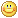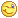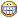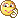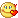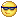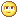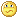نظر خصوصی
مشخصات شما ذخیره شود ؟ [حذف مشخصات] [شکلک ها]کد امنیتی
اطلاعات کاربری
 نام کاربری : رمز عبور :
• فراموشی رمز عبور؟
• لینک دوستان
آرشیو
آمار سایت
• کل مطالب : 155
• کل نظرات : 0
• افراد آنلاین : 1
• تعداد اعضا : 4
• آی پی امروز : 16
• آی پی دیروز : 17
• بازدید امروز : 120
• باردید دیروز : 81
• گوگل امروز : 0
• گوگل دیروز : 1
• بازدید هفته : 364
• بازدید ماه : 336
• بازدید سال : 36,510
• بازدید کلی : 157,562
• کدهای اختصاصی